angle of inclination. Slope is denoted by the letter m. Therefore, If the axes are in their conventional positions, a line, such as AB in figure 1-4, view A, that slopes upward and to the right will have a positive slope. A line, such as CD in figure 1-4, view B, that slopes downward and to the right will have a negative slope."> Inclination and SlopeCustom SearchINCLINATION AND SLOPE The angle of inclination, denoted by the Greek let­ter alpha (), as portrayed in figure 1-4, views A and B, is the angle formed by the line crossing the X axis and the positively directed portion of the X axis, such that 0 ° << 180'. The slope of any line is equal to the tangent of its angle of inclination. Slope is denoted by the letter m. Therefore,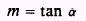If the axes are in their conventional positions, a line, such as AB in figure 1-4, view A, that slopes upward and to the right will have a positive slope. A line, such as CD in figure 1-4, view B, that slopes downward and to the right will have a negative slope. Since the tangent of a is the ratio of PM to P1M, we can relate the slope of line AB to the points P1 and P2 as follows: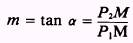Designating the coordinates of P1 as (x1,y1) and those of P2 as (x2,y2), we recall that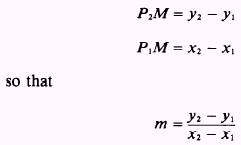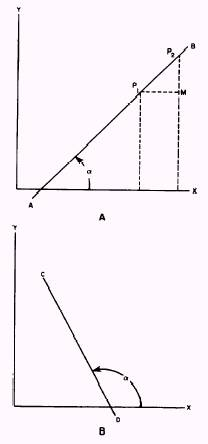Figure 1-4.-Angles of inclination. The quantities (x2 - x1) and (y2 - y1) represent changes that occur in the values of the x and y coordinates as a result of the change from P2 to P1 on line AB. The symbol used by mathematicians to represent an increment of change is the Greek letter delta (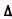). Therefore,x means "the change in x" andy means "the change in y." The amount of change in the x coordinate, as we change from P2 to P1, is x2- x1. Therefore,and likewise,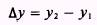We use this notation to express the slope of line AB as follows: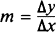EXAMPLE: Find the slope of the line connecting P2(7,6) and P1(-1,-4). SOLUTION:Note that the choice of labels for P1 and P2 is strictly arbitrary. In the previous example, if we had chosen the point (7,6) to be P1 and the point (-1, - 4) to be P2, the following results would have occurred:Notice that this solution yields the same result as the last example. A slope of 5/4 means that a point moving along this line would move vertically + 5 units for every horizontal movement of + 4 units. This result is consistent with our definition of positive slope; that is, sloping upward and to the right. If line AB in figure 1-4, view A, was parallel to the X axis, y1 and y2 would be equal and the difference (y2 - y1) would be 0. Therefore,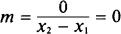We conclude that the slope of a horizontal line is 0. We can also reach this conclusion by noting that angle a (fig. 1-4, view A) is 0 ° when the line is parallel to the X axis. Since the tangent of 0° is 0, then m=tan0°=0 The slope of a line that is parallel to the Y axis becomes mean­ingless. The tangent of the angle a increases indefinitely as a approaches 90°. We sometimes say that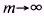(m approaches in­finity) when a approaches 90°.Integrated Publishing, Inc. - A (SDVOSB) Service Disabled Veteran Owned Small Business